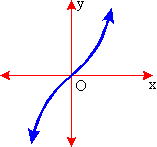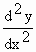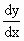Home MonkeyNotes Printable Notes Digital Library Study Guides Study Smart Parents Tips College Planning Test Prep Fun Zone Help / FAQ How to Cite New Title Request

5.11 Stationary Points(1) The condition that f ' (x) i.e.= 0 at x = c, for f (x) should be maximum or minimum is necessary but it is not sufficient

Consider f (x) = x3

Now= 3x2= 0 at x = 0

Also,= 6x then at x = 0,= 0

y = x3

Henceat x = 0 is neither negative nor positive

hence at x = c is neither a maximum nor a minimum.

(2) The maxima and minima of f(x) are also known as 'turning values' of f(x) sincechanges its sign from positive to negative or from negative to positive.

They are also known as "extrema or extreme values."Your browser does not support the IFRAME tag.

(3) The values of the function for= 0 are known as critical values or stationary values since f (x) is neither increasing nor decreasing at these values (points). It is a value or point at which the gradientis zero.

Note that a stationary point is not necessarily a turning point. It is clear from the above example given in (1). At (0, 0)= 0 and the x - axis is the tangent there. However, (0, 0) is not a turning point because f (x) continues to increase when passing through it. The origin is stationary since the curve y = f (x) is momentarily stationary there.

Index

5.1 Tangent And Normal Lines
5.2 Angle Between Two Curves
5.3 Interpretation Of The Sign Of The Derivative
5.4 Locality Increasing Or Decreasing Functions 5.5 Critical Points
5.6 Turning Points
5.7 Extreme Value Theorem
5.8 The Mean-value Theorem
5.9 First Derivative Test For Local Extrema
5.10 Second Derivative Test For Local Extrema
5.11 Stationary Points
5.12 Concavity And Points Of Inflection
5.13 Rate Measure (distance, Velocity And Acceleration)
5.14 Related Rates
5.15 Differentials : Errors And Approximation